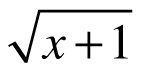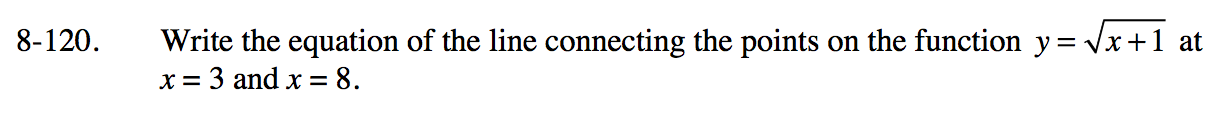### Home > PC3 > Chapter 8 > Lesson 8.3.3 > Problem8-120

8-120.

Write the equation of the line connecting the points on the function y =at x = 3 and x = 8. Homework Help ✎Determine the y-coordinates for each of the points.

Calculate the slope of the line connecting the two points.

Use a point from step 1 and the slope from step 2 to write the equation of the line.